As in Section 8.2.2, potential functions can be used to represent feedback plans, assuming that a local operator is developed that works for continuous state spaces. In the discrete case, the local operator selects an action that reduces the potential value. In the continuous case, the local operator must convert the potential function into a vector field. In other words, a velocity vector must be defined at each state. By default, it will be assumed here that the vector fields derived from the navigation function are not necessarily normalized.

Assume that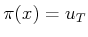is defined for all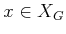, regardless of the potential function. Suppose that a potential function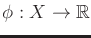has been defined for which the gradient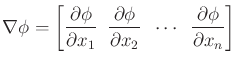(8.40)

exists over all of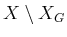. The corresponding feedback plan can then be defined as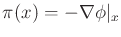. This defines the local operator, which means that the velocity is taken in the direction of the steepest descent of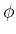. The idea of using potential functions in this way was proposed for robotics by Khatib [525,526] and can be considered as a form of gradient descent, which is a general optimization technique.

It is also possible to work with potential functions for which the gradient does not exist everywhere. In these cases, a continuous-space version of (8.4) can be defined for a small, fixed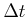as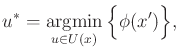(8.41)

in which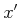is the state obtained by integrating velocityfromfor time. One problem is thatshould be chosen to use the smallest possible neighborhood around. It is best to allow only potential functions for whichcan be made arbitrarily small at everywithout affecting the decision in (8.41). To be precise, this means that an infinite sequence of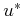values can be determined from a sequence ofvalues that converges to 0. A potential function should then be chosen to ensure after some point in the sequence,, exists and the samecan be chosen to satisfy (8.41) asapproaches 0. A special case of this is if the gradient ofexists; the infinite sequence in this case converges to the negative gradient.

A potential function,, is called a navigation function if the vector field that is derived from it is a solution plan. The optimal cost-to-go serves as an optimal navigation function. If multiple vector fields can be derived from the same, then every possible derived vector field must yield a solution feedback plan. If designed appropriately, the potential function can be viewed as a kind of ski slope'' that guides the state to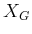. If there are extra local minima that cause the state to become trapped, thenwill not be reached. To be a navigation function, such local minima outside ofare not allowed. Furthermore, there may be additional requirements to ensure that the derived vector field satisfies additional constraints, such as bounded acceleration.

Example 8..17 (Quadratic Potential Function)   As a simple example, suppose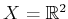, there are no obstacles, and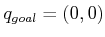. A quadratic function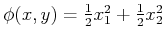serves as a good potential function to guide the state to the goal. The feedback motion strategy is defined as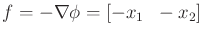, which is the inward vector field shown in Figure 8.5b.

If the goal is instead at some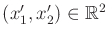, then a potential function that guides the state to the goal is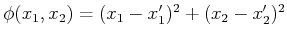.Suppose the state space represents a configuration space that contains point obstacles. The previous functioncan be considered as an attractive potential because the configuration is attracted to the goal. One can also construct a repulsive potential that repels the configuration from the obstacles to avoid collision. Let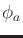denote the attractive component and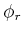denote a repulsive potential that is summed over all obstacle points. A potential function of the form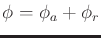can be defined to combine both effects. The robot should be guided to the goal while avoiding obstacles. The problem is that it is difficult in general to ensure that the potential function will not contain multiple local minima. The configuration could become trapped at a local minimum that is not in the goal region. This was an issue with the planner from Section 5.4.3.

Steven M LaValle 2020-08-14